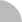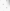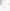##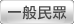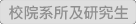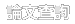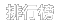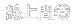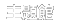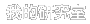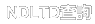(3.238.173.209) 您好！臺灣時間：2021/05/16 05:55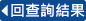### 詳目顯示:::

: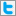Twitter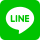•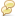被引用:0
•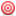點閱:119
•評分: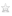•下載:22
•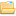書目收藏:0
 我們研究下列Logistic擴散問題之分支曲線和其正解個數{█((u''(x)+λ[ru(1-u/q)-u/(1+mu+u^2 )]=0,-10, m≥1, 和 λ > 0 是一個分支參數。則我們在 (λ‚‖u‖_∞ )平面上研究分支曲線的圖形，並且確定問題正解之個數。關鍵字：Logistic擴散問題；解之個數；正解；時間映射。
 We study exact multiplicity and bifurcation curves of positive solutions for a diffusive logistic problem{█((u''(x)+λ[ru(1-u/q)-u/(1+mu+u^2 )]=0,-10, m≥1, and λ > 0 is a bifurcation parameter. Then on the (λ‚‖u‖_∞ )-plane, we study shapes of bifurcation curves, and hence we are able to determine the multiplicity of positive solutions.
 中文摘要 i英文摘要 ii誌 謝 iii目 錄 iv1 Introduction 12 Main results 43 Lemmas 74 Proof of Main results 9Reference 14
  K.-J. Huang, Exact multiplicity of postive solutions for a boundary vale problem with a quartic polynomial. Unpublished master's thesis, University of Tainan, Tainan, Taiwan, 2015. T. Laetsch, The number of solutions of a nonlinear two point boundary value problem, Indiana Univ. Math. J. 20 (1970) 1--13. S.-H. Wang and N. D. Kazarinoff , Bifurcation and stability of positive solutions of a two-point boundary value problem, J. Austral. Math. Soc. Ser. A 52 (1992), 334--342. S.-H. Wang and N. D. Kazarinoff , Bifurcation of steady-state solutions of a scalar reaction-diffusion equation in one space variable, J. Austral. Math. Soc. Ser. A 52 (1992), 343--355. T.-S. Yeh, Classification of bifurcation diagrams for a multiparameter diffusive logistic problem with Holling type-IV functional response, J. Math. Anal. Appl. 418 (2014) 283-304.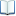電子全文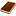國圖紙本論文推文當script無法執行時可按︰推文 網路書籤當script無法執行時可按︰網路書籤 推薦當script無法執行時可按︰推薦 評分當script無法執行時可按︰評分 引用網址當script無法執行時可按︰引用網址 轉寄當script無法執行時可按︰轉寄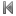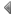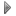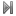top
 1 某一邊界值問題分支曲線完整分類 2 包含恰有三個正零根的非線性函數p-拉普拉斯問題正解的分支曲線 3 包含恰有一個正零根的非線性函數p-拉普拉斯問題正解的確切個數 4 包含四次多項式邊界值問題正解的確切個數 5 包含四次多項式邊界值問題正解的分支曲線 6 一類三次非線性正定問題全分枝性及確切正解個數 7 數類p-拉普拉斯多重參數分枝問題全分枝性及確切正解個數之研究 8 一維推廣Ambrosetti-Brezis-Cerami問題解集合的結構

 無相關期刊

 無相關點閱論文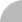簡易查詢 | 進階查詢 | 熱門排行 | 我的研究室